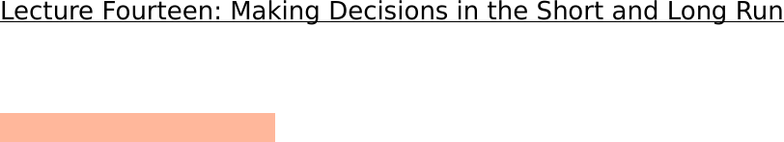Class Notes (1,100,000)
CA (630,000)
York (40,000)
ECON (2,000)
ECON 1000 (500)
Lecture 4

# ECON 1000 Lecture Notes - Lecture 4: Sunk Costs, Average Cost, Marginal Cost

Department
Economics
Course Code
ECON 1000
Professor
George Georgopoulos
Lecture
4

Page:
of 4Lecture Fourteen: Making Decisions in the Short and Long Run
November 17, 2011
Decision Time Frames
The firm makes man decisions to achieve its main objective: profit
maximization
Some decisions are critical to the survival of the firm
-Some decisions are irreversible or very costly to reverse
Other decisions are easily reversed and are less critical to the survival of the firm,
but they still influence the profit.
All decisions can be placed into two time frames
-The short run
-The long run
The Short Run
The short run is a time frame in which the quantity of one or more resources
used in production is fixed.
-The time that it takes to change the quantity of the short run is considered to be
the short run
For most firms, the capital, called the firms plant, is fixed in the short run.
Other resources used by the firm (such as labour, raw materials an energy) can
be changed in the short run.
Short run decisions are easily reversed.
The Long Run
The long run is a time frame in which the quantities of all the resources,
including plant size, can be varied
Long run decisions are not easily reversed
A sunk cost is a cost incurred by the firm and cannot be changed
-If a firms’ plant has no resale value, the amount paid for it is the sunk cost
-Start up costs of opening a business also are sunk costs because you do not
get that money back when you decide to close the business
Sunk costs are irrelevant to a firm’s current decisions
Short Run Technology Constraint
To increase output in the short run, a firm must increase the amount of labour
employed.
There are three concepts to describe the relationship between output and the
quantity of labour employed:
1. Total Product
2. Marginal Product
3. Average Product
Product Schedules
Total Product is the total output produced in a given period
The marginal product of labour is the change in total product that results from
a one unit increase in the quantity of labour employed, with all other outputs
remaining the same
The average product of labour is equal to total product divided by the quantity
of labour employed
As the quantity of labour employed increases,
- Total product increases
- Marginal product initially increases, but eventually decreases
- Average product initially increases, but eventually decreases
One of the reasons to explain this is that during this time, the firms capital
resources (such as machinery or technology) is remaining the same. Therefore
there might not be enough equipment compared to the amount of people
working.
As marginal and average product increases, marginal cost decreases. There is
an inverse relationship between the two.
Increasing marginal returns arise from increased specialization and division
of labour.
Diminishing marginal returns arises from the tact that employing additional
units of labour mens each worker has less access to capital and less space in
which to work.
Short Run Cost
To produce more output in the short tun, the firm must employ more labour, which
means that it must increase its costs.
We describe the way a firms costs change as total product change by using
three concepts and three types of cost curve:
-Total Cost (this includes the fixed cost)
-Marginal Cost
-Average Cost
Total Cost - the cost of all the resources used
-Total Fixed Cost: the cost of the firms fixed inputs. FIxed costs do not change
with a change in output
-Total Variable Cost: the costs of the firms variable inputs. Variable costs
change with the amount of output.
TC = TFC+TVC
Marginal Costs
Marginal Cost is the increase in total cost that results from a one unit increase
in output
Over the output range with increasing marginal returns, marginal cost falls as
output increases
Over the output range with diminishing marginal returns, marginal cost ruses as
output decreases.
Average Costs
Average cost measures can be derived from each of the total cost measures:
Average fixed costs: is the total fixed cost per unit of output
Average variable cost: is the total variable cost per unit of output
Average total cost is the total cost per unit.